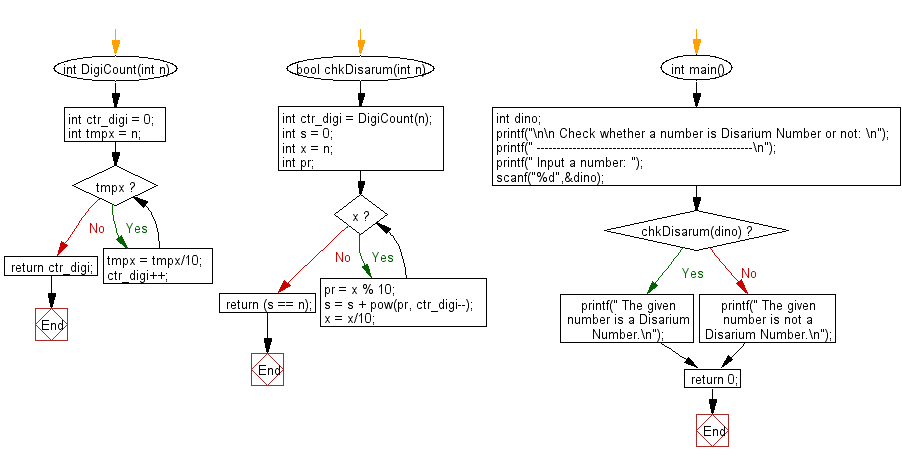﻿ C Program: Check whether a number is Disarium or not - w3resource# C Exercises: Check whether a number is Disarium or not

## C Numbers: Exercise-15 with Solution

Write a program in C to check whether a number is Disarium or not.

Test Data
Input a number: 135

Sample Solution:

C Code:

``````# include <stdio.h>
# include <math.h>
# include <stdlib.h>
# include <stdbool.h>

int DigiCount(int n)
{
int ctr_digi = 0;
int tmpx = n;
while (tmpx)
{
tmpx = tmpx/10;

ctr_digi++;
}
return ctr_digi;
}
bool chkDisarum(int n)
{
int ctr_digi = DigiCount(n);
int s = 0;
int x = n;
int pr;
while (x)
{
pr = x % 10;
s = s + pow(pr, ctr_digi--);
x = x/10;
}
return (s == n);
}
int main()
{

int dino;
printf("\n\n Check whether a number is Disarium Number or not: \n");
printf(" ------------------------------------------------------\n");
printf(" Input a number: ");
scanf("%d",&dino);

if( chkDisarum(dino))
printf(" The given number is a Disarium Number.\n");
else
printf(" The given number is not a Disarium Number.\n");
return 0;
}
```
```

Sample Output:

``` Input a number: 135
The given number is a Disarium Number.
```

Pictorial Presentation:Flowchart:C Programming Code Editor:

What is the difficulty level of this exercise?

Test your Programming skills with w3resource's quiz.

﻿

## C Programming: Tips of the Day

Why do C and C++ compilers allow array lengths in function signatures when they're never enforced?

It is a quirk of the syntax for passing arrays to functions.

Actually it is not possible to pass an array in C. If you write syntax that looks like it should pass the array, what actually happens is that a pointer to the first element of the array is passed instead.

Since the pointer does not include any length information, the contents of your [] in the function formal parameter list are actually ignored.

Ref : https://bit.ly/3fhlvdH